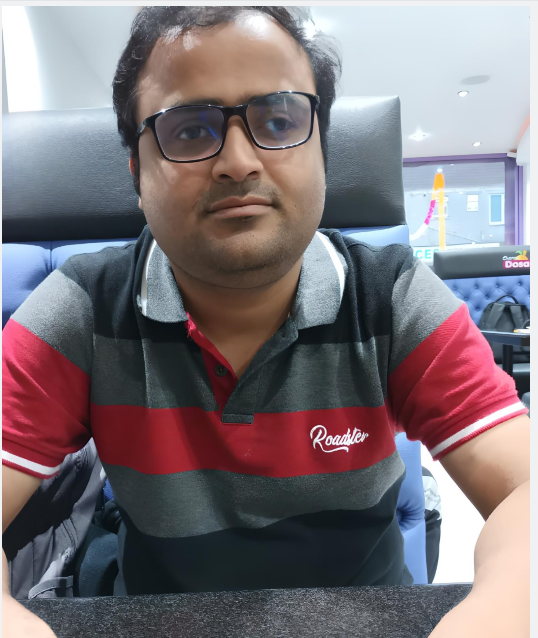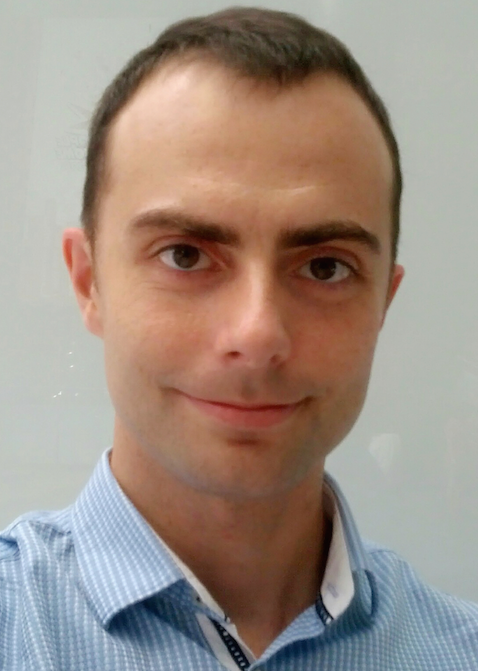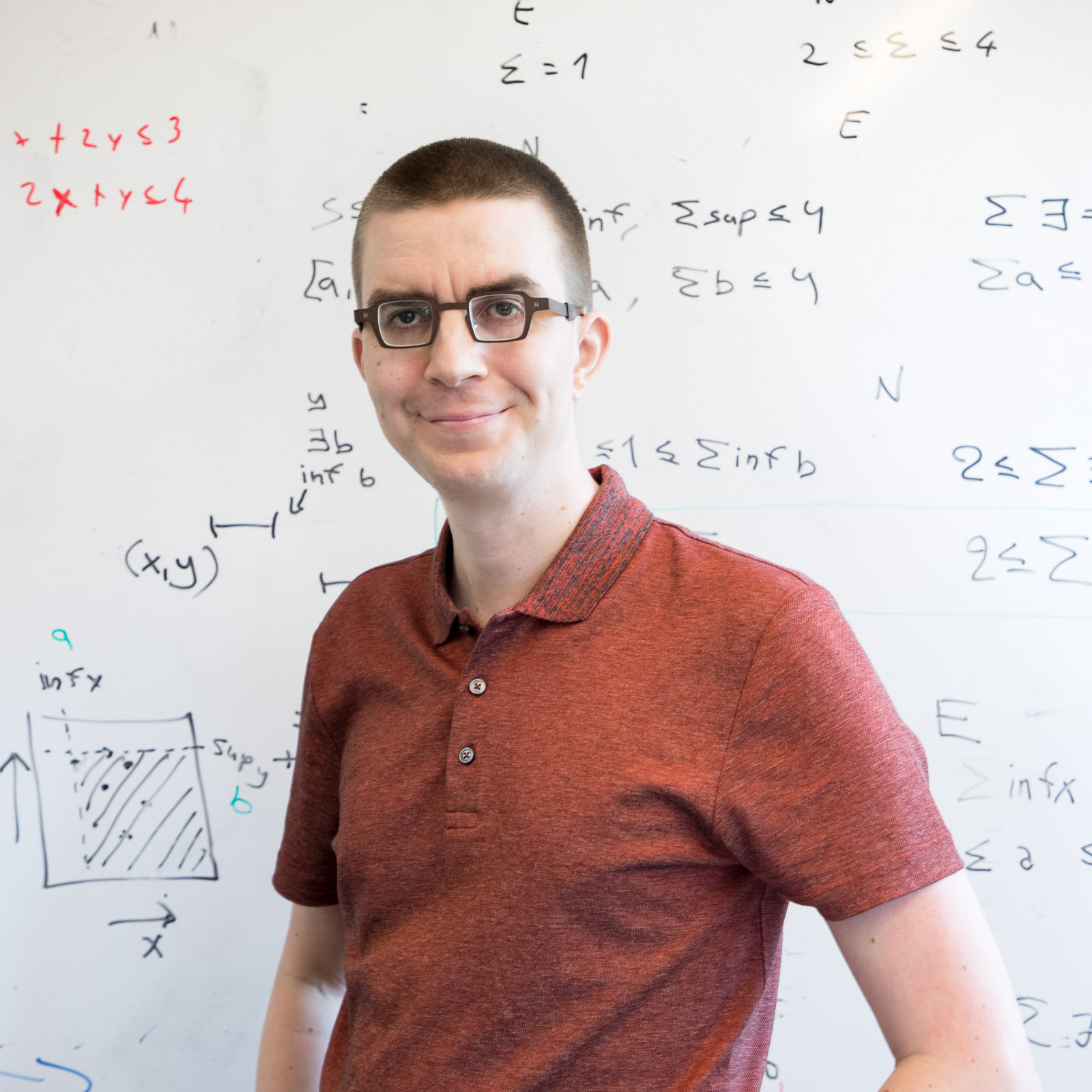Algorithms for Massive Graphs
AMG 2023

AMG2023 is the second iteration of a one-day workshop that focuses on algorithms for big data and large graphs.

AMG 2023 will be held on Friday, 13th October 2023, and will be co-located with DISC 2023. The workshop will be chaired by Jara Uitto.

# Schedule

All times in Central European Summer Time (CEST), GMT+2.

TBA: AMG programme
10:00 - 11:00 Talk 1 Gopinath Mishra: On Coloring problems in MPC and Congested Clique
11:20 - 12:20 Talk 2 Peter Robinson: Distributed Graph Algorithms in the Vertex-Partition Model
14:00 - 15:00 Talk 3 Talya Eden: Sampling an Edge in Sublinear Time Exactly and Optimally
15:00 - 16:00 Talk 4 Jukka Suomela: Low-degree graphs, sparse matrices, and low-bandwidth networks
16:00 Workshop ends

# Invited SpeakersGopinath Mishra

National University of Singapore (NUS)

Gopinath Mishra is a Post Doctoral Fellow at the National University of Singapore (NUS) hosted by Yi-Jun Chang. He received his Ph. D. from Indian Statistical Institute where he was advised by Arijit Bishnu and Arijit Ghosh. Prior to joining NUS, he was a Post Doctoral Fellow at the University of Warwick hosted by Artur Czumaj.

Title: On Coloring problems in MPC and Congested Clique

Abstract: Graph coloring problems are among the most extensively studied problems in the area of distributed graph algorithms. The inherent interaction between local and global aspects of the coloring problems makes them representative for a variety of problems in distributed setting. In the distributed graph coloring problem, we are given an undirected graph node of the graph such that no edge is monochromatic. The most fundamental graph coloring problem in distributed computing is $(\Delta+1)$-coloring which can be easily solved by a sequential greedy algorithm, but the interaction between local and global aspects of graph coloring creates some non-trivial challenges in a distributed setting. The problem has been used as a benchmark to study distributed symmetry breaking in graphs, and it is at the very core of the area of distributed graph algorithms. A generalization of $(\Delta+1)$-coloring is the (degree+1)-list coloring (D1LC) problem which is more challenging to solve in the distributed models. In this talk, we discuss the results on coloring problems in Massively Parallel Computation (MPC) and distributed computing with focus on some recent developments on (degree+1)-list coloring (D1LC) problem.Peter Robinson

Augusta University

Peter Robinson is an Associate Professor at Augusta University. Previously, he was an Assistant Professor at City University HK and at McMaster University. His research interests are in the area of distributed graph algorithms, fault-tolerant algorithms, and complexity lower bounds. His work received the best paper award at SSS 2008 and ICDCN 2013. He served as the general chair of PODC 2019 and on the PODC steering committee from 2017 to 2019.

Title: Distributed Graph Algorithms in the Vertex-Partition Model

Abstract: In this talk, we consider the vertex-partition model, where the vertices of an input graph are partitioned among $k$ players who communicate via message passing, and the goal is to output the solution to a graph problem. In the extreme case where $k = n$, the model coincides with standard distributed computing models. For $k \ll n$, on the other hand, it can be viewed as a theoretical model for iterative graph processing systems that follow the "think like a vertex" paradigm, which includes, e.g., Pregel and Giraph. We will survey algorithmic results for concrete problems such as maximal matching, as well as discuss the technical difficulties of showing lower bounds that, similarly to the distributed graph sketching model, emanate from the fact that every edge may be known by two players.Talya Eden

Bar Ilan University

Talya Eden is an Assistant Professor at the computer science department at Bar Ilan University. She works on sublinear-time and randomized graph algorithms for huge data sets. Her work focuses on theoretical and applied graph parameter estimation in sublinear-time, as well as graph algorithms in other related areas, such as the streaming model, the massively parallel computation model, and learning-augmented algorithms.

Talya completed her PhD at the School of Electrical Engineering at Tel Aviv University under the supervision of Prof. Dana Ron, and then continued on to a postdoctoral fellowship with the Foundations of Data Science Institute at MIT and the Computer Science department at Boston University, where she was hosted by Prof. Ronitt Rubinfeld, Prof. Pioter Indyk, and Prof. Sofya Raskhodnikova. Talya has won several awards for her work, including the EATCS Distinguished Dissertation Award in Theoretical Computer Science, a Rothschild Postdoctoral Fellowship, a Schmidt Postdoctoral Award, a Ben Gurion University postdoctoral fellowship, a Weinstein Graduate Studies Prize, and the Azrieli Fellows Scholarship.

Title: Sampling an Edge in Sublinear Time Exactly and Optimally

Abstract: Sampling edges from a graph in sublinear time is a fundamental problem and a powerful subroutine for designing sublinear-time algorithms. Suppose we have access to the vertices of the graph and know a constant-factor approximation to the number of edges. An algorithm for pointwise $\varepsilon$-approximate edge sampling with complexity $O(n/\sqrt{\varepsilon m})$ has been given by Eden and Rosenbaum [SOSA 2018]. This has been later improved by T\v{e}tek and Thorup [STOC 2022] to $O(n \log(\varepsilon^{-1})/\sqrt{m})$. At the same time, $\Omega(n/\sqrt{m})$ time is necessary. We close the problem by giving an algorithm with complexity $O(n/\sqrt{m})$ for the task of sampling an edge exactly uniformly.Jukka Suomela

Aalto University

Jukka Suomela is Associate Professor in the Department of Computer Science at Aalto University, Finland. His work focuses on the theoretical foundations of distributed and parallel computing, with particular emphasis on the concept of locality. He was the PC chair of DISC 2019 and SIROCCO 2016 and one of the local chairs of ALGO 2018, he is serving in the EATCS council, and he is currently the chair of the DISC steering committee. He has received the FOCS 2019 best paper award and DISC 2012 and 2017 best paper awards, as well as a number of teaching awards.

Title: Low-degree graphs, sparse matrices, and low-bandwidth networks

Abstract: Matrix multiplication is a key primitive for solving many graph problems. For example, as soon as we have an efficient parallel algorithm for matrix multiplication, we can use it to detect and count triangles in a graph. Indeed, this kind of algebraic algorithms outperform the best combinatorial algorithms for many graph problems.

Matrix multiplication has been studied extensively e.g. in the congested clique model, and one of the surprises here is that improvements in centralized sequential algorithms for matrix multiplication also imply improvements in parallel algorithms for matrix multiplication in the congested clique model.

However, the congested clique model is not the right model to study sparse graphs. For example, triangle counting in bounded-degree graphs corresponds to a matrix multiplication task in which we are multiplying uniformly sparse matrices. In sufficiently sparse graphs, there is a trivial algorithm that solves the problem in constant time in the congested clique model. But of course this does not mean that counting triangles in huge graphs is a trivial problem in practice; the congested clique model is simply way too powerful. In particular, the trivial algorithm can abuse the large bandwidth that we have available in the model. In this talk we will look at what happens when we study sparse matrix multiplication in a more reasonable setting: low-bandwidth networks.

This talk is based on joint work with Keren Censor-Hillel, Chetan Gupta, Juho Hirvonen, Petteri Kaski, Janne H. Korhonen, Christoph Lenzen, Ami Paz, Jan Studený, Hossein Vahidi, and many others.